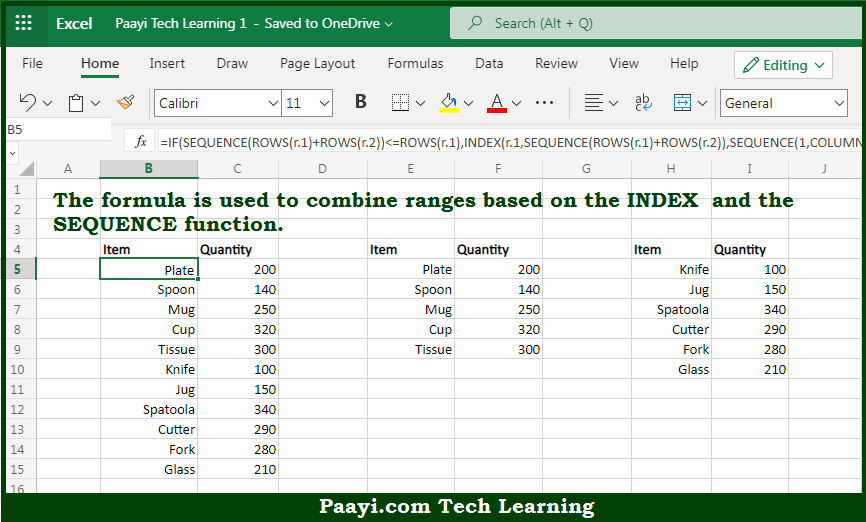# Learn How to How to Combine Ranges in Microsoft Excel

Written by | 0 Comments | 592 Views

In this article, you will learn how to evaluate things in Dynamic Arrays with formulas in Microsoft Excel using a single/combination(s) of functions. You will also know How to Combine Ranges and see the generic formula.

How to Combine Ranges in Microsoft Excel

The main purpose of this formula is to combine ranges. Here we will learn how to combine ranges with a formula in the workbook in Microsoft Excel. That implies, with the help of a formula based on the INDEX and SEQUENCE function you can able to combine ranges. So, with the help of this formula, you can able to combine ranges with a formula in the workbook in Microsoft Excel.

General Formula to Combine Ranges

=IF(SEQUENCE(ROWS(r.1)+ROWS(r.2))<=ROWS(r.1),INDEX(r.1,SEQUENCE(ROWS(r.1)+ROWS(r.2)),SEQUENCE(1,COLUMNS(r.1))),INDEX(r.2,SEQUENCE(ROWS(r.1)+ROWS(r.2))-ROWS(r.1),SEQUENCE(1,COLUMNS(r.1))))

The Explanation for the Combine RangesSo we know that with the help of the given formula above you can able to combine ranges. Here we will learn how to combine ranges with a formula in the workbook in Microsoft Excel. As we know that the IF function is used to compare all values in the row index sequence with the row count for range 1. In case a row index value is less than or equal to the count of the rows in range 1 (5), the INDEX function is used to fetch rows from range 1. So, with the help of this formula, you can able to combine ranges with a formula in the workbook in Microsoft Excel.